Next: 6. Related Work Up: Toward Cost-Sensitive Modeling for Previous: 4. Cost-Sensitive Modeling

Subsections

# 5. Experiments

Our experiments use data that were distributed by the 1998 DARPA Intrusion Detection Evaluation Program. The data were gathered from a military network with a wide variety of intrusions injected into the network over a period of 7 weeks. The details of our data mining framework for data pre-processing and feature extraction is described in our previous work . We used 80% of the data for training the detection models. The training set was also used to calculate the precision of each rule and the threshold value for each class label. The remaining 20% were used as a test set for evaluation of the cost-sensitive models.

## 5.1 Measurements

We measure expected operational and consequential costs in our experiments. The expected average operational cost per event over the entire test set is defined as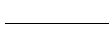. In all of our reported results, OpCost(e) is computed as the sum of the feature computation costs of all unique features used by all rules evaluated until a prediction is made for event e. If any level 3 features (of cost 100) are used at all, the cost is counted only once. This is done because a natural optimization of rule evaluation is to compute all statistical and temporal features in one iteration through the event database.

For each event in the test set, its CCost is computed as follows: the outcome of the prediction (i.e., FP, TP, FN, TN, or misclassified hit) is used to determine the corresponding conditional cost expression in Table 2; the relevant RCost, DCost, and PCost are then used to compute the appropriate CCost. The CCost for all events in the test set are then summed to measure total CCost as reported in Section 5.2. In all experiments, we setand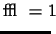in the cost model of Table 2. Settingcorresponds to the optimistic belief that the correct response will be successful in preventing damage. Settingcorresponds to the pessimistic belief that an incorrect response does not prevent the intended damage at all.

 --- +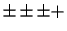--+ OpCost 128.70 48.43 42.29 222.73 48.42 47.37 %rdc N/A 56.68% 67.14% N/A 78.26% 78.73%

## 5.2 Results

In all discussion of our results, we use +, - andto represent +freq, -freq and un-ordered rulesets, respectively. A multiple model approach is denoted as a sequence of these symbols. For example, -- represents a multiple model where all rulesets are -freq.

Table 3 shows the average operational cost per event for a single classifier approach (R4 learned as - or +) and the respective multiple model approaches (, -- or, --+). The first row below each method is the average OpCost per event and the second row is the reduction (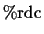) by the multiple model over the respective single model,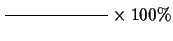. As clearly shown in the table, there is always a significant reduction by the multiple model approach. In all 4 configurations, the reduction is more than 57% and --+ has a reduction in operational cost by as much as 79%. This significant reduction is due to the fact thatare very accurate in filtering normal events and a majority of events in real network environments (and consequently our test set) are normal. Our multiple model approach computes more costly features only when they are needed.

 Model Format --- +--+ CCost 25776 25146 25226 24746 24646 24786 [0pt] Cost Sensitive %rdc 87.8% 92.3% 91.7% 95.1% 95.8% 94.8% CCost 28255 27584 27704 27226 27105 27258 [0pt] Cost Insensitive %rdc 71.4% 75.1% 74.3% 77.6% 78.5% 77.4% %err 0.193% 0.165% 0.151% 0.085% 0.122% 0.104%

CCost measurements are shown in Table 4. The Maximalloss is the cost incurred when always predicting normal, or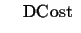. This value is 38256 for our test set. The Minimal loss is the the cost of correctly predicting all connections and responding to an intrusion only when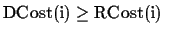. This value is 24046 and it is calculated as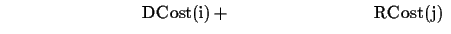. A reasonable method will have a CCost measurement between Maximal and Minimal losses. We define reduction as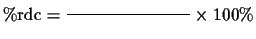to compare different models. As a comparison, we show the results of both cost sensitive'' and cost insensitive'' methods. A cost sensitive method only initiates a response if, and corresponds to the cost model in Table 2. A cost insensitive method, on the other hand, responds to every predicted intrusion and is representative of current brute-force approaches to intrusion detection. The last row of the table shows the error rate (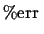) of each model.

As shown in Table 4, the cost sensitive methods have significantly lower CCost than the respective cost insensitive methods for both single and multiple models. The reason is that a cost sensitive model will only respond to an intrusion if its response cost is lower than its damage cost. The error rates for all 6 models are very low (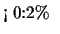) and very similar, indicating that all models are very accurate. However, there is no strong correlation between error rate and CCost, as a more accurate model may not necessarily have detected more costly intrusions. There is little variation in the total CCost of single and multiple models in both cost-sensitive and cost-insensitive settings, showing that the multiple model approach, while decreasing OpCost, has little effect on CCost. Taking both OpCost and CCost into account (Tables 3 and 4), the highest performing model is --+.

It is important to note that all results shown are specific to the distribution of intrusions in the test data set. We can not presume that any distribution may be typical of all network environments.Next: 6. Related Work Up: Toward Cost-Sensitive Modeling for Previous: 4. Cost-Sensitive Modeling